﻿ Numerical Simulation of a Spiral Wall

### Numerical Simulation of a Spiral Wall

A. Z.DELLILOPEN ACCESSPEER-REVIEWED

## Numerical Simulation of a Spiral Wall

Institut de Maintenance et de Sécurité Industrielle, IMSI, Université d'es-senia,Laboratoire. Mécanique .Appliquée USTO, Oran, ALGERIE

### Abstract

This work presents a study of the thermal characteristics of a turbulent flow of a forced convection along various geometrical configurations: Smooth wall, wall corrugated in spiral, corrugated wall. The effects of pitch/diameter ratio and rib height to diameter ratio made it possible to develop this impact of this geometrical form (sometimes corrugated, sometimes spiral) in the intensification of the heat transfer. The physical process of increase in the heat transfer comes owing to the fact that the spiral shape of the tube contributes to break the formed boundary layer and to allow the continual replacement of the fluid meadows of the solid wall. The numerical results obtained prove that this corrugated form or into spiral intensifies the heat transfer thanks to model SST for Reynolds numbers varying from 5000 to 55000, Indeed when the pitch and the depth of the rib increases from P/dh = 0.18, e/dh = 0.02 to P/dh = 0.27, e/dh = 0.06. The ratio of the numbers of Nusselt increases with the rise in the step and the depth of the notch. Several explanations can be advanced, among these last, the spirals are the cause of the presence of the viscous effects, which slow down the flow in the notch of the spiral (broken boundary layer). The low depth of the notch, going from 0.02 up to 0.04 does not allow a significant thermal intensification, on the other hand for the strong values of the notch (0.05 to 0.06) the presence of turbulence supports good Nusselt. We notes that this last increases with the amplitude of the undulation up to the value of 0.05H. Beyond the value of the amplitude, the number of Nusselt tends towards the constant value.

### At a glance: Figures

12345
Prev Next

• Z.DELLIL, A.. "Numerical Simulation of a Spiral Wall." American Journal of Industrial Engineering 1.2 (2013): 5-11.
• Z.DELLIL, A. (2013). Numerical Simulation of a Spiral Wall. American Journal of Industrial Engineering, 1(2), 5-11.
• Z.DELLIL, A.. "Numerical Simulation of a Spiral Wall." American Journal of Industrial Engineering 1, no. 2 (2013): 5-11.

 Import into BibTeX Import into EndNote Import into RefMan Import into RefWorks

### 1. Introduction

Many researchers were interested in experimental studies in order to quantify the impact of the modification of the geometry of the walls on the intensification of the heat transfer. Among these authors, Hudson , Chauve and Schistel  which approached the experimental part for compressible fluids, incompressible and for various geometries, amongst other things: rectangular. In 1993, Saniei and Dini , tested the characteristics of a heat transfer in turbulent mode for a corrugated channel. Experimentally and in this field, we can note the work of Hsu and Kennedy , which was completed in a control, inside which the wall presented spirals. The experiment was led with air and water. The results obtained are completely modified compared to smooth control. The principal cause of these modifications of the profile speed and the thermal transfer being variation of the section due to the effect of the curves of the internal wall and the presence of the currents return behind each peak. By carrying out an experimental part, in which, it locally measures a certain quantity of waste heat by the plate, and the friction coefficient against the wall. Many of these experiments were carried out in permanent mode  and non stationary mode . A model of numerical simulation to design tubes rib for condensers of water heating at summer developed by Rousseau and al . It was especially noted that corrugated surface or spiral form, for a mode of steady flow, modifies the results of turbulence appreciably. The measurement of certain terms which characterize the turbulent movement on a plane wall was the subject of many studies of the laminar case while passing by the transient with the turbulent one. The use of the hot wire anemometer indeed made it possible to explain much case. We will quote the study of the boundary layer along a plane plate by Klebanoff, Schubaeur and Clauser . Other parameters, such as the simplicity of construction, machining, maintenance are also significant to take into account in the stage of the design of these thermal systems. In the presence of modification of geometries (ribs and amplitude), the acceleration which undergoes the fluid with the collar and the deceleration which is followed from there to the hollow translates on the profiles speed by successive effects the convergent ones and the divergent comparable ones with those obtained by J Nikuradse . They noted that the maximum of the number of local Nusselt is located at the upstream of each undulation, whereas the minimum of the number of local Nusselt was placed downstream, at a short distance of the peak of each undulation. Hudson and others  began an experimental research on the water run-off in a rectangular channel whose lower wall was carried out with corrugated detachable Plexiglas plates. The non stationary currents return was highlighted in experiments at the same time by visualization in water and hot wire in the air by M.P.Chauve . The reduction in the non stationary currents return to high Reynolds numbers seems to have to be explained by the increase in the heat transfer.

The numerical resolution of the problems arising for the determination of the thermal characteristics of the flows on plates was the subject of many publications. Recently, the results obtained in experiments were confirmed numerically by the use of the model k-ε de Jones and Launders . J.Gosse and R.Schistel  seem to be among the authors studied numerically the thermal convection on these walls of a vertical vapour generator to a master key. Various approaches were used to study the influence of the spirals low depths on these turbulent flows. In addition to the experimental part, R.Schiestel and M.P.Chauve  presented a numerical part. This modelling is primarily based on the principle of turbulent viscosity, by introducing the notion of turbulent Prandtl which consists in calculating heat flows by the assumption of turbulent thermal diffusivity. The results obtained thus for the second time showed that the traditional values obtained for the wall planes are completely modified because of the variations of section (concave and convex left alternation), from where the presence of movements return behind each peak. While taking as a starting point the work by Launder and Spalding  concerning the incompressible flows, C.Mari, D.Jeandel and J.Mathieu  presented a simple model which numerically treats the development of the compressible layer boundary with a heat transfer. Patel and al.  presented a new calculation based on algorithm SIMPLER and the model k-ε, by using the profile of one tenth of a poise (into laminar) like initial conditions to start the resolution of the Navier-stokes equations. Richmond and al  proceeded to the same study with a mode of flow. The effects of the geometry and the parameters of turbulence were the subject of a numerical study for a laminar flow on a undulated wall in two dimensions by G.Tanga and G.Vittori . In recent numerical1 simulations, Wang and Vanka  determined the thermal rate of transfer for a periodic flow in a corrugated geometrical configuration. They observed that average Nusselt for a channel undulated in mode of permanent flow, was slightly larger than that of the parallel channel with walls. However, in the transitory mode, the heat transfers being improved of approximately 2.5 times. In stationary mode, the factor of Friction for a channel with corrugated walls was approximately twice more significant than that of a parallel channel with walls, and remains almost constant in the transitory mode. Moreover, the comparison of the predicted results of an undulated wall with those of a right channel without undulations shows that the average Nusselt number increases until a value criticizes is reached at the place where the amplitude of undulation is maximum. The effect of the amplitude of the undulation as well on the heat transfer as on the pressure losses was largely documented. This type of study by its high degree of accuracy is regarded as the rival of the experimental studies in several cases. Wang and Vanka  noticed, through a numerical study of the steady flows and non-stationary on corrugated walls that the rate of heat transfer is slightly high. The transition takes place for a Reynolds number equal to 180 approximately for the same geometrical configuration. In addition to the dynamic field, the our characteristic is work: consists in solving the fields of flow and thermics starting from the equations of RANS (Equations of Reynolds and Navier-stokes averages) with modelSST(Shear-Stress-Transport). The performances of these corrugated and spiral forms are much better than those of the plane forms . This corrugated and spiral geometry have a sinusoidal form obtained by a succession of arc. These forms thus cause of:

• To limit the thermal stresses on these walls due to their differential expansions compared to the grill

• To increase their surface for a given distance

• To increase the convection on both sides these plates

• To allow the presence of secondary movements of the fluid due to the alternate changes of the curves to increase the pressure losses.

For the three cases of geometrical configuration smooth tube (see Figure 1), spiral corrugated tube (see Figure 2), corrugated tube (see Figure 3) we used an incompressible, permanent and turbulent fluid. To ensure itself of the establishment of the thermal mode, dynamic and periodic,

Our study of this flow will be carried out far from the entry and the exit. The physical properties of the fluid are taken constant. As Boundary conditions we took at the entry a profile uniform speed, an intensity of turbulence equal to 5%. At the exit, the gradient of the pressure being null with a perfect adherence with the wall.

• Smooth wall

• Undulated wall in the shape of spiral

• Corrugated wall

According to the Figure 2, we varied the depth of the rib as well as the step.

### 2. Mathematical Formulation and Description of the Problem

For the three cases of geometrical configuration smooth tube, spiral corrugated tube (see Figure 1), corrugated tube (see Figure 2) we used an incompressible, permanent and turbulent fluid. To ensure itself of the establishment of the thermal mode, dynamic and periodic, our study of this flow will be carried out far from the entry and the exit. The physical properties of the fluid are taken constant. As Boundary conditions we took at the entry a profile uniform speed, an intensity of turbulence equal to 5%. At the exit, the gradient of the pressure being null with a perfect adherence with the wall Smooth wall

• Undulated wall in the shape of spiral

• Corrugated wall

According to the Figure 1, we varied the depth of the rib as well as the step. All the variables are without dimensions, maybe with the height H or the mean velocity U. The all of the data is recorded in the form of dated such as: data 01, data 02 until data10, for the 10 values of position taken along a length of undulation (Table 2), such as (Figure 2):

#### Table 2 Four various positions of undulation

The undulation of the corrugated wall is described by:(1)

Where am is the amplitude of the undulation andis the wavelength.

### 3. Mathematical Model

The incompressible flows of fluids are controlled by the Navier-Stokes equations. These equations, which express physical laws of conservation, result from the laws of Newton of the movement.

They connect speed and the pressure in each point of the flow. Four unknown functions must be given: three components the speed and the pressure. For a problem of heat transfer, the temperature is the fifth variable to be determined. The flow being turbulent, two other quantities will be calculated. Initially, it is useful to present the final mathematical model written in a curvilinear frame of reference generalized marrying perfectly the curved borders of the field of calculation and allowing a natural installation of the boundary conditions.

3.1. Model of Turbulence: SST (Shear Stress Transport)

The simulations were processed using the CFX 11.0 software from ANSYS, Inc. In the solver package, the solution of the Reynolds Averaged Navier-Stokes Equations is obtained by using finite volume method with a body-fitted structured grid. A cell-centered layout is employed in which the pressure, turbulence and velocity unknowns share the same location. The momentum and continuity equations are coupled through a pressure correction scheme and several implicit first and second order accurate schemes are implemented for the space and time discretizations. In the present computation, convection terms written in convective form are discredited with a third order upwind-biased scheme. The turbulence closure is done with the help of the k-shear stress transport (SST) turbulence model of Menter . The main advantage of this model is its capability to be applied in the high Reynolds number region as well as in the near wall regions. The strategy is based on a blend of the k-model of Wilcox  in the near wall region and the standard k -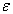model  further away from the wall. Due to the high capability behaviour of the k -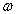model near the wall, the SST model does not need explicit damping when approaching the wall. The well-known dependence of free stream values ofis eliminated by the blend of the k -model. The details of Reynolds Averaged Navier Stokes equations as well as the SST turbulence model are not given here since they are well documented in the literature.

The formulation of the model of SST is as follows:(2)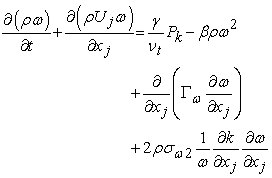(3)(4)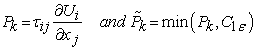(5)

The coefficients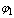 andof the model are functions of:(6)

Where the coefficients of the modeland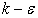model are respectively equal (see Table 3).

### 4. Grid

The grids carried out with software ANSYS-ICEM are of hexahedral type and tetrahedral. The grid is carried out so that it is refined on the level of the rib and of the undulation (see Figure 3) for a validation of the model of turbulence to knowing the SST. Several grids were used and tested for the three configurations(see Table 4). The table which follows watches the characteristics of this grid. The grids described in the table above were studied and tested, to choose the suitable grid. The results of this study will be presented in the chapter results and discussion.

### 5. Results and Discussion

5.1. Validation

The objective of this work is the validation of the turbulence model of the code quoted before, through a numerical study of a turbulent flow above a smooth and corrugated wall. It is a question of comparing our numerical results carried out with code CFX-11 (SST) with those of the experiment of Dittus and Boettler through the Nusselt number(Figure 4) and Friction Factor (Figure 5).We show starting from several tests, initially on a plane wall that the models give coherent results. For the SST, the grid must be very refined near the wall to collect the maximum of data, for the various values of the reports/ratios of depth and step varying from P/dh= 0.18, 0.22, 0.27 and e/dh=0.02, 0.04, 0.06. All These models rest primarily on the principle of turbulent viscosity.

5.2. Nusselt and coefficient of friction

The results which we present relate primarily to the impact of a modification of the geometry of a wall in the improvement of the heat transfer. Indeed, through these results, we notes that compared to a smooth wall, we note a clear improvement of the heat transfer of the loss Equal pressure.

5.2.1. Case of a Corrugated-Spiral Tube

We have carried out our calculations for various values not going from 0.18 up to the maximum value 0.27 and various depths going of 0.02 until 0.06. The variation of the number of local Nusselt along a spiral-circle in the area most developed compared to the depth of the spiral-circle is shown on Fig 6. The latter illustrate although on the one hand the Nusselt number increases value with the fur as the step and the depth increase (P/dH = 0.27 and e/dH = 0.06), and in addition the Nusselt number believes according to the Reynolds number. It is noticed especially that the Nusselt number increase almost value and passes from the value 200 pour (P/dH = 0.18 and e/d H = 0.02) to 350 for (P/dH = 0.27 and e/dH = 0.06). All in all, an increase in the value of the amplitude is accompanied by an increase by the Nusselt numberand losses pressure. This modification of the wall supports the diffusion, then the turbulent mixture. To recreate effects being able to improve the heat transfer in such a geometrical configuration, it is necessary to limit the evolution of the value of the amplitude to take into account that the effect of loss pressure is caused (Figure 7).

5.2.1.1. Geometrical Influence on Profiles of the Coefficient of Friction

The distributions of the coefficients of friction are represented on Figure 7. It is noted that the profile of the coefficient of friction decrease by value for all the depth and step values. We also observe that this coefficient of friction increases for the weak modes of flow and decrease for great Reynolds numbers. For the smooth wall, the coefficient of friction is constant. By comparing the results of the coefficients of friction obtained by the experiment of Dittus and those obtained numerically by the SST, the same reduction in this coefficient is indicated. With the fur as the depth and the step increase value, turbulence increases thus supporting the presence of a strong depression.

5.3.1. Ratio of the Rate of Heat Transfer

On Figure 8, we notices that almost for all the modes of flow, the report/ratio of the numbers of Nusselt increases with the rise in the step and the depth of the depth of the depth of the rib. Several explanations can be advanced, among these last, the spirals are the cause of the presence of the viscous effects which slow down the flow in the of depth of the depth of the rib of the spiral. But because of the low depth of the rib, going from 0.02 up to 0.04, the variation is not very significant. The flow near the wall for the Reynolds number significant and the strong values of the depth of the depth of the rib (0.06) supports turbulence, thus under boundary layer is broken supporting the heat transfer.

5.4. Case of a Corrugated Tube

We observe on the Fig above flatness and a compressing of the boundary layer thermal for the amplitude of 0.05H. It results a contact tightened between the wall and the fluid, from where a good level of thermal transfer (Figure 9). The variation of the number of local Nusselt along an undulation in the area most developed compared to the amplitude of the undulation is noted on Figure 10. The latter illustrates well that the Nusselt numberis constant and independent of the mode of flow for null amplitude (case of the plane wall). Locally Nusselt decreases by value to convergent of the undulation and increases at the top of this one. We observe a light shift of the Nusselt numbertowards the downstream when the value of the amplitude takes the values from 0.02H to 0.1H. Therefore the average Nusselt marks a maximum for the undulated wall compared to the plane wall by undergoing a light shift towards the downstream of the undulation. On the whole, an increase in the value of the amplitude is accompanied by an increase by the Nusselt number and pressure losses. The undulation of the wall supports the diffusion, then the turbulent mixture. On this Figure 10, we notes that this number of average Nusselt increases with the amplitude of the undulation up to the value of 0.05H. Beyond the value of the amplitude of 0.05H, the Nusselt number tends towards the constant value.

To recreate effects being able to improve the heat transfer in such a geometrical configuration (Figure 11 a and b), it is necessary to limit the evolution of the value of the amplitude to take into account the effect of the occasioned loss pressure. The distributions of the coefficients of friction are represented on Figure 12 a and b, on Figure 12 a, we notes that from the 7 ième undulation, the profile of the coefficient of friction becomes periodic. It is observed that for only one undulation the coefficient of friction increases at the top and decrease with the hollow of the latter. Figure 12 b shows the variation of the distribution of the coefficients of friction, located by the two positions of the hollow (10.25) and the top (10.75) along an undulation for the various amplitudes. For the plane wall (null amplitude), the coefficients of friction are constant. By comparing the results of the coefficients of friction of the plane wall (am=0) and the undulated wall, we indicate a clear reduction in this coefficient in the hollow and an increase in its value at the top of the undulation for the whole of the amplitudes (Figure 12). Except for the smallest value of amplitude (am= 0.02H), where, the distribution of this coefficient is strongly deformed. We note a light increase in its value to the hollow of the undulation. For the low amplitudes, where the depression is weak (weak recirculation). With the fur as the amplitude, therefore turbulence with the hollow increase thus supporting the presence of a strong depression.

5.5. Comparison of the Various Geometrical Configurations

We observe on this Figure 13, the same evolution of the Nusselt number for the three configurations. Only and compared to the smoothes tube, and for all the modes of flow, the undulated tube into spiral form and the corrugated tube present a better heat transfer for the same step, the same value of the rib and the same value of amplitude. From Re=30000, a significant profit in heat transfer is observed compared to the smooth tube.

### 6. Conclusion

The numerical results presented in this study prove that the alternation of the undulation contributes to a consequent heat exchange, in spite of the loss pressure which results from it. The effects of this improvement are observed through the Nusselt number, which increases value compared to that of a plane wall. Even the structure of the flow was modified thanks to this geometry. The low depth of the notch, going from 0.02 up to 0.04 does not allow a significant thermal intensification, on the other hand for the strong values of the notch (0.05 to 0.06) the presence of turbulence supports good Nusselt. We notes that this last increases with the amplitude of the undulation up to the value of 0.05H. Beyond the value of the amplitude, the number of Nusselt tends towards the constant value. On the other hand, the strong values of the notch support the increase in the pressure losses. The results for the friction coefficient note that growth in this ratio from 0.03 to 0.05. It is clear that these modifications of these walls modify the structure of turbulence near the wall. Only these low value the pitch and the depth of the notch could not be well explored and detailed by this model SST. To conclude this study, the model of turbulence SST noted the increase in the thermal transfer and the loss pressure compared to the plane wall. At equal loss pressure, the undulated wall in spiral presents a good heat transfer for the maximum of pitch and depth of the rib compared to the other walls (smooth, corrugated-spiral and corrugated).

However, it is not a handicap because it was noted that the profit in heat is carried out even in the case of a slightly higher loss pressure compared to that of the plane wall. It is clear that these modifications of these walls modify the structure of turbulence near the wall. In prospect, other models of the second order will be able to justify this intensification of heat for the spiral and corrugated walls0.

### References

  Hudson.J.D. 1993. ’The effect of a wavy boundary on turbulent flow’. PhD,University of Illinois, Urbana, IL.In article CrossRef  Schiestel, R.; Chauve, M.P.. 1982. ’Influence d’ondulation de faible amplitude sur une convection turbulente en conduite axisymétrique’, Communication au 5ième congrès français de mécanique. Marseille.In article CrossRef  Saniei, N.; Dini S.. 1993. ‘Heat transfer characteristics in a wavy walled channel’, J.Heat Transfer 115, 788-792.In article CrossRef  Hsu, S.T; Kennedy, J.F.. 1971.’Turbulent flow in wavy pipes’, J.Fluid Mech.47, 481-502.In article CrossRef  Tanda ,G.; Vittori, G. (1996). ‘Fluid flow and heat transfer in a two dimensional wavy chanel, Heat Mass Transfer 31, 411-418.In article  Rousseau, P.G.; Van Eldik, M., G.P.Greyvenstein. 2003. ‘Detailed simulation of fluted tube water heating Condensers’, International Journal of refrigeration 26, 232-239.In article CrossRef  Klebanoff.P.S, Schubaeur G.B.1955. ‘Investigation of separation of the turbulent Boundary layer’ N.A.C.A. tech.Note n° 2133.In article  Nikuradse J. 1930.’Turbulente stromung in nicht kreisformigen Rohren’.  Ind. Arch 1.In article  Hudson J,; Dykhno L ,; Hanratty. 1996. ‘Turbulent production in flow over wavy wall‘, Exp. Fluids 20, 257-65.In article CrossRef  Jones W. P.,; Launder B. E. 1972.’ Prediction of Relaminarisation with a Two Equation Turbulence Model‘, Int. Journal of Heat and Mass Transfer, 15,310-314.In article CrossRef  Gosse J.,; Schiestel R.. 1976. ‘Convection dans les tubes ondulés’, Revue Générale de thermique, 172.In article  Launder B. E.; Spalding D. B.. 1972.’ Mathematical models of turbulence Academic Press’, London and N.-Y.In article PubMed  Mari C.,; Jeandel D.,; Mathieu J. .1975. ‘Méthode de calcul de la couche limiteTurbulente compressible avec transfert de chaleur’, Int. J. Heat .Mass transfer, Vol.19, 893-899.In article CrossRef  Patel V.C.,; Rodi W.,; Scheuerer G.. 1985. ‘Turbulence models for near wall and low Reynolds number flows’: A review. AIAA Journal, 23, 1308-1319.In article CrossRef  Richmond.M.C,V.; Patel C.. 1991.’Convex and concave surface curvatures effects in Wall bounded turbulent flow’, AIAA.J.vol 29, 895-902.In article  Tyndall Chon J;Yoon J . Y. 1991.’Turbulent Flow in a Channel with a Wavy wall’, Journal of Fluids Engineering, Vol. 113, pp. 579-586.In article CrossRef  Wang,G.; Vanka S. P..1995. ’Convective Heat Transfer in Periodic Wavy Passages’, Int. J. Heat Mass Transfer, Vol. 38, N°.17, 3219-3230.In article CrossRef  Pethkool, S.; Eiasaard, S; Kwankaomeng, S.; Promvonge P. 2011. ’Turbulent HeatTransfer enhancement in a heat exchanger using helically corrugated tube’, Int Communications in heat and mass transfer, Vol 38, 340-347.In article CrossRef  Menter, F.R.1994. ’Two-equation eddy-viscosity turbulence models for engineering applications’. AIAA-Journal, 328, pp. 269-289.In article  Wilcox, D.C. 1993. ’Turbulence Modeling for CFD’. DCW Industries, Inc., La Canada, CA.In article